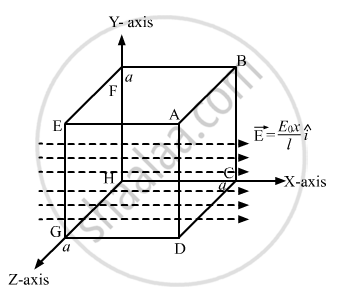Department of Pre-University Education, KarnatakaPUC Karnataka Science Class 12
Share

# The electric field in a region is given by → E = E 0 x l → i . Find the charge contained inside the cubical volume bound by the surfaces - Physics

ConceptElectric Field Introduction of Electric Field

#### Question

The electric field in a region is given by vec"E" = ("E"_0 "x")/"l"  vec"i".

Find the charge contained inside the cubical volume bound by the surfaces

x =0, x =a, y=0, y=a, z=0 and z=a. Take

"E"_0 = 5 xx 10^3 "N""C"^-1 , "l" =2 "cm" " and" " a" = 1 "cm"

#### Solution

Given:
Electric field strength, vec"E" = ("E"_0"x")/"l"  hat"i"

Length, l = 2 cm
Edge of the cube, a = 1 cm

E0 =5.0 × 103 N/CIt is observed that the flux passes mainly through the surfaces ABCD and EFGH. The surfaces AEFB and CHGD are parallel to the electric field. So, electric flux for these surfaces is zero.
The electric field intensity at the surface EFGH will be zero.
If the charge is inside the cube, then equal flux will pass through the two parallel surfaces ABCD and EFGH. We can calculate flux passing only through one surface. Thus,

vec"E" = ("E"_0"x")/"l"  hat"i"

At EFGH, x = 0; thus, the electric field at EFGH is zero.
At ABCD, x = a; thus, the electric field at ABCD is   vec"E" = ("E"_0"a")/"l"  hat "i"

The net flux through the whole cube is only through the side ABCD and is given by

phi = vec"E" . vec "A" = (("E"_0"a")/"l"  hat"i") . ("a" hat"i") = ("E"_0"a"^2)/"l".

Net flux = phi  =( 5 xx 10^3)/( 2 xx 10^-2) xx ( 1 xx 10 ^-2)^2  "N""m"^2 // "C" = 25 "Nm"^2 // "C"

Thus the net charge,

q = ∈0 phi

q = 8.85 × 10 -12 × 25

q =22.125 ×10-13

q =2.2125 ×10 -12 C

Is there an error in this question or solution?

#### APPEARS IN

Solution The electric field in a region is given by → E = E 0 x l → i . Find the charge contained inside the cubical volume bound by the surfaces Concept: Electric Field - Introduction of Electric Field.
S